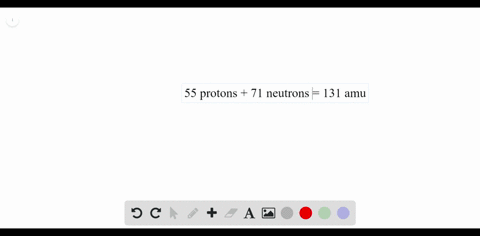### Xenon An isotope of xenon has an atomic number of…

00:18
Problem 72University of Miami
Problem 68

Mercury An isotope of mercury has 80 protons and 120
neutrons. What is the mass number of this isotope?

Mass number $=200$ amu.

## Discussion

You must be signed in to discuss.

## Video Transcript

So now we'll work on problem 68 from chapter four. This problem were asked about Ah, an isotope of mercury, which has 80 protons in 120 Notre neutrons. And so for this isotope, what would the mass number B so mass number is equal to crew thons plus new Schranz. So in this case, that would be 80 protons plus 120 new treatments, so our mass number for this mercury isotope would be 200.